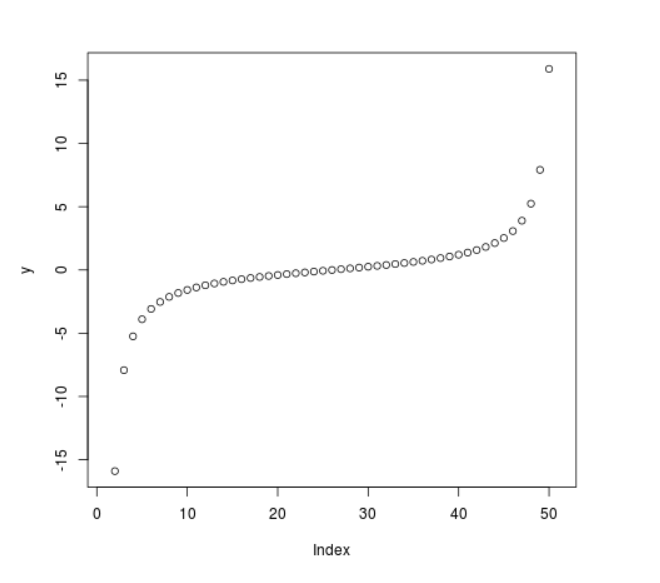# Compute the value of Cauchy Quantile Function in R Programming – qcauchy() Function

• Last Updated : 25 Jun, 2020

`qcauchy()` function in R Language is used to calculate the value of cauchy quantile function. It also creates a density plot of cauchy quantile function.

Syntax: qcauchy(vec, scale)

Parameters:
vec: x-values for cauchy function
scale: Scale for plotting

Example 1:

 `# R Program to compute value of``# cauchy quantile function`` ` `# Creating vector for x-values``x <``-` `seq(``0``, ``1``, by ``=` `0.2``)`` ` `# Apply qcauchy() Function``y <``-` `qcauchy(x, scale ``=` `4``)``y`

Output:

```      -Inf -5.505528 -1.299679  1.299679  5.505528       Inf
```

Example 2:

 `# R Program to compute value of``# cauchy quantile function`` ` `# Creating vector for x-values``x <``-` `seq(``0``, ``1``, by ``=` `0.02``)`` ` `# Apply qcauchy() Function``y <``-` `qcauchy(x, scale ``=` `1``)`` ` `# Plotting the graph``plot(y)`

Output:My Personal Notes arrow_drop_up